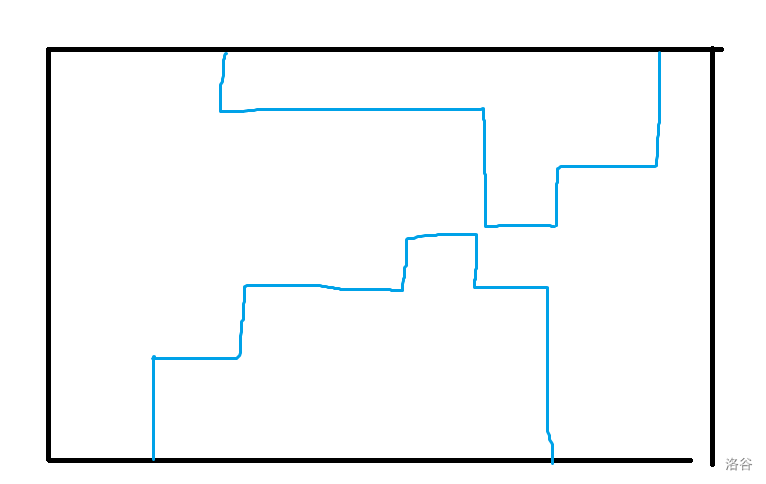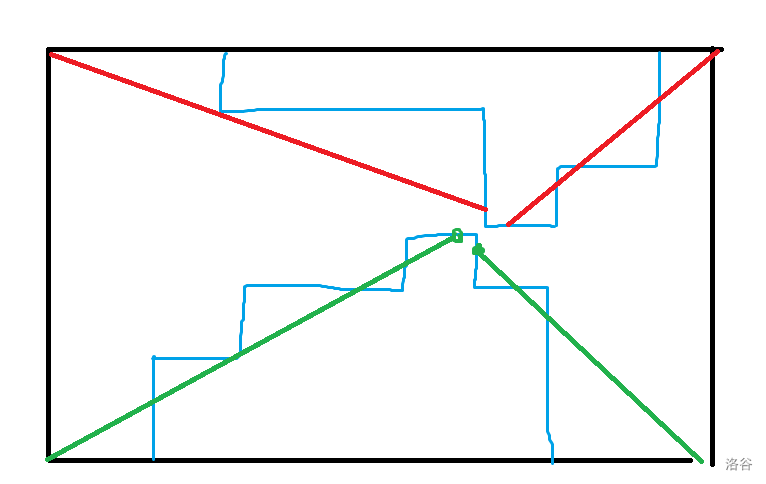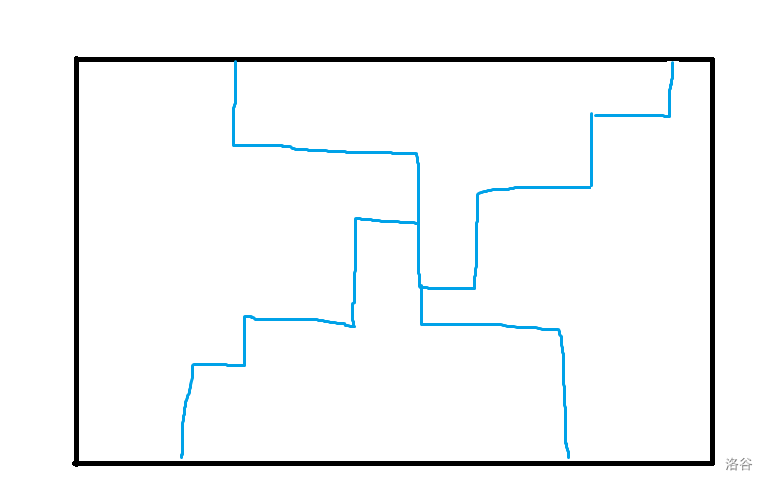zoukankan      html  css  js  c++  java
• # Codeforces Round #712 (Div. 1) 题解

## A.Balance the Bits

题目描述

点此看题

解法

其实就是让两个括号序列是合法的。

一看就是构造题，还是考虑最终答案有哪些限制，很重要的一点就是左括号数量和右括号数量相等(0) 不会对相等关系造成影响，(1) 的话就必须要有偶数个，而且一半是左括号一半是右括号。

现在我们贪心地尽量放左括号即可（每个时刻都要满足左括号(geq)右括号），(1) 的话就前一半放左括号，后一半放右括号，(0) 的话就看哪边左括号少就把左括号给哪边就行。

``````#include <cstdio>
const int M = 200005;
{
int x=0,f=1;char c;
while((c=getchar())<'0' || c>'9') {if(c=='-') f=-1;}
while(c>='0' && c<='9') {x=(x<<3)+(x<<1)+(c^48);c=getchar();}
return x*f;
}
int T,n,m,f,c1,c2,cnt,a[M];char s[M];
signed main()
{
while(T--)
{
c1=c2=cnt=m=f=0;
scanf("%s",s+1);
for(int i=1;i<=n;i++)
if(s[i]=='1') m++;
if(m%2) {puts("NO");continue;}
//construct
for(int i=1;i<=n;i++)
{
if(s[i]=='0')
{
if(c1>c2) c1--,c2++,a[i]=0;
else c1++,c2--,a[i]=1;
}
else
{
cnt++;
if(cnt<=m/2) c1++,c2++,a[i]=0;
else c1--,c2--,a[i]=1;
}
if(c1<0 || c2<0) f=1;
}
if(f) {puts("NO");continue;}
puts("YES");
for(int i=1;i<=n;i++)
if(s[i]=='0')
printf("%c",a[i]==0?')':'(');
else
printf("%c",a[i]==0?'(':')');
puts("");
for(int i=1;i<=n;i++)
if(s[i]=='0')
printf("%c",a[i]==0?'(':')');
else
printf("%c",a[i]==0?'(':')');
puts("");
}
}
``````

## B. 3-Coloring

题目描述

点此看题

解法

又是构造题，我一直想着从左到右从上到下去填，但是发现总是有奇奇怪怪的问题，这种方法不行的原因就是因为没有全局视野，这道题位置明明可以随便放，为什么要这么局限呢？

如果没有 ( t Alice)( t Bob) 肯定会选择对这个棋盘黑白染色，现在有了 ( t Alice)，但是又给了你蓝色，所以我们的策略是当 ( t Alice) 把你逼进绝路时我们使用蓝色来活命，可以构造处下列策略：

• 如果 ( t Alice) 办蓝色，那么我们继续对原图黑白染色。
• 如果 ( t Alice) 办白色，我们看能不能填黑色，如果不能填我们就把蓝色填到白色的地方，这时候四周都是黑色，不会冲突。
• 如果 ( t Alice) 办黑色，我们看能不能填白色，如果不能填我们就把蓝色填到黑色的地方。

记录一下白色和黑色的位置，就可以 (O(n^2)) 通过此题。

``````#include <cstdio>
#include <iostream>
using namespace std;
const int M = 10005;
#define pii pair<int,int>
#define mp make_pair
{
int x=0,f=1;char c;
while((c=getchar())<'0' || c>'9') {if(c=='-') f=-1;}
while(c>='0' && c<='9') {x=(x<<3)+(x<<1)+(c^48);c=getchar();}
return x*f;
}
int n,x,l1,l2;pii a[M],b[M];
void work1(int t)
{
printf("%d %d %d
",t,a[l1].first,a[l1].second);
fflush(stdout);l1--;
}
void work2(int t)
{
printf("%d %d %d
",t,b[l2].first,b[l2].second);
fflush(stdout);l2--;
}
signed main()
{
for(int i=1;i<=n;i++)
for(int j=1;j<=n;j++)
{
if((i+j)&1) a[++l1]=mp(i,j);
else b[++l2]=mp(i,j);
}
for(int i=1;i<=n*n;i++)
{
cin>>x;
if(x==3)
{
if(l1) work1(1);
else work2(2);
}
if(x==1)
{
if(l2) work2(2);
else work1(3);
}
if(x==2)
{
if(l1) work1(1);
else work2(3);
}
}
}
``````

## C. Travelling Salesman Problem

题目描述

点此看题

解法

首先不要被起点 (1) 给影响了，其实经过所有点恰好一次相当于走一个环，环的话起点就无意义了。

本题最妙的一步是对代价做一个简单的转化，把同源的东西放在一起是重要的思想（一家人就应该完完整整的）：

[max(c_i,a_j-a_i)=c_i+max(0,a_j-a_i-c_i) ]

可以按 (a_i) 排序，我们从左到右扫，维护 (a_i+c_i) 的最大值，如果此时 (a_j) 大于 (a_i+c_i) 就更新答案，并且此时跳一定比以后跳更优，可以把对答案的贡献写出来，你发现现在就跳会赚 (c_j) 元钱，然后跳到 (n) 之后就可以暴力往回跳了。

也可以线段树优化 (dp)，设 (dp[i]) 表示走到 (i) 的最小花费，那么不难写出转移：

[dp[i]=min(min_{a_jleq a_i+c_i}dp[j],(min_{a_j>a_i+c_i}dp[j]+a_j)-(a_i+c_i)) ]

两种做法的时间复杂度都是 (O(nlog n)) 的。

``````#include <cstdio>
#include <algorithm>
using namespace std;
const int M = 100005;
#define ll long long
{
int x=0,f=1;char c;
while((c=getchar())<'0' || c>'9') {if(c=='-') f=-1;}
while(c>='0' && c<='9') {x=(x<<3)+(x<<1)+(c^48);c=getchar();}
return x*f;
}
int n,mx;ll ans;
struct node
{
int a,c;
bool operator < (const node &R) const
{
return a<R.a;
}
}s[M];
signed main()
{
for(int i=1;i<=n;i++)
{
s[i]=node{a,c};ans+=c;
}
sort(s+1,s+1+n);
for(int i=1;i<=n;i++)
{
mx=max(mx,s[i].a+s[i].c);
if(mx<s[i+1].a) ans+=s[i+1].a-mx;
}
printf("%lld
",ans);
}
``````

## D.Flip the Cards

题目描述

(n) 个卡牌，正面写着 (a_i) 反面写着 (b_i)，问能否将若干个卡牌翻面，再经过任意排序后使得正面数字单调递增，反面数字单调递减，如果不能输出 (-1)，否则输出最小翻面次数。

(nleq 200000)保证 ([1,2n]) 每个数恰好出现一次

解法

惯用的思考技巧是考虑最终答案的形式，正面单增，反面单减，有什么性质呢？结合 ([1,2n]) 每个数恰好出现一次的性质，正面的一段前缀有 ([1,n]) 中的数，反面的一段后缀会有 ([1,n]) 中的数（指排序后的结果），最重要的是前缀和后缀一定没有相交的部分

由这个性质可以引出一个结论，每张牌一定一面的数字在 ([1,n])，另一面的数字在 ((n,2n]) 中，可以用这个判断无解。

继续考虑这个简化了的问题，你发现最终答案正面的最小数一定对应的反面的最大数，反面的最小数一定对应着正面的最大数，并且正面的反面感性上是独立的，对于其他数也有类似的大小关系。可以把卡牌按 ([1,n]) 的数字排序，设排序后数字 (i) 对应的 ((n,2n]) 的数字是 (f[i])，可以从 (f[i]) 里面划分出两个并集为全集，互不相交的单减子序列，那么第一个子序列可以按顺序放到正面（从前面开始放），第二个子序列可以按顺序放到反面（从后面开始放），这样就构造出了最终答案。

现在的问题是求子序列划分的最小代价。先考虑怎么判断有无解吧，我们用两个栈维护单减子序列，把当前元素加入栈顶元素较小的栈是最可能有解的（但不一定是最优的），这种放法可能不优的源头就是本来两个栈都可以放的时候做了不好的选择（比如原来是反面放入了第一个栈，会有 (1) 的消耗），那么我们要考虑什么时候两个栈都可以放，并且后面放的时候能保证合法，发现只有这一种可能：

[min_{jleq i}f[j]>max_{j>i}f[j] ]

如果满足这个条件显然是可以顺便放的（后面形成的子序列接在哪一个上面都可以），但如果不满足这个条件能否随便放呢？假设我们已经把前面满足这个条件的都断开了，而且 (f[i]) 能随便放说明 (f[i]) 就是前缀最小值，而如果随便放导致和 (i-1) 的前缀最小值错开的话，就会无解，所以只能和 (i-1) 的前缀最小值放在同一个栈中（因为 (i-1) 的前缀最小值也是小于 (max_{j>i}f[j]) 的），证毕。

其实根据证明我们也可以得到一些算法的启发，我们根据上面的判断条件把原序列划分成若干个段，每一段都有唯一的划分方式，就是贪心地加到栈顶较小的那个栈里面去，但是要注意第一个序列和第二个序列可以整体换位，也就是对于 ([l,r]) 算出来的答案是 (c)，那么 (r-l+1-c) 这种答案也是合法的（不考虑这个样例都过不了），时间复杂度 (O(n))

这道题我觉得可以线段树优化 (dp)，但是有点小地方没想通，有兴趣的你可以自己想一想

``````#include <cstdio>
#include <iostream>
#include <stack>
using namespace std;
const int M = 400005;
const int inf = 1e8;
{
int x=0,f=1;char c;
while((c=getchar())<'0' || c>'9') {if(c=='-') f=-1;}
while(c>='0' && c<='9') {x=(x<<3)+(x<<1)+(c^48);c=getchar();}
return x*f;
}
int n,ans,f[M],b[M],pre[M],suf[M];
//f[i] stand for value i(<=n) -> f[i] (>n)
//b[i] stand for the initial state
int work(int l,int r)
{
int p=inf,q=inf,c=0;
for(int i=l;i<=r;i++)
{
if((p<q || f[i]>q) && f[i]<=p)//add into the first stack
{
p=f[i];
c+=(b[i]!=0);
}
else if(f[i]<=q)//add into the second stack
{
q=f[i];
c+=(b[i]!=1);
}
else return 0;
}
ans+=min(c,r-l+1-c);
return 1;
}
signed main()
{
for(int i=1;i<=n;i++)
{
if(x>y) swap(x,y),b[x]=1;//stupid mistake
if(x<=n && y<=n) {puts("-1");return 0;}
f[x]=y;
}
pre=inf;
for(int i=1;i<=n;i++)
pre[i]=min(pre[i-1],f[i]);//prefix min
for(int i=n;i>=1;i--)
suf[i]=max(suf[i+1],f[i]);//suffix max
for(int i=1,j;i<=n;i=j+1)
{
j=i;
while(pre[j]<=suf[j+1]) j++;
if(!work(i,j)) {puts("-1");return 0;}
}
printf("%d
",ans);
}
``````

## E.2-Coloring

题目描述

点此看题

解法

首先要思考答案可能长什么样子，有一种情况是这样的：其中被蓝色路径框起来的部分涂上了蓝色。总结一下这种情况就是一个在下一个在上，向外扩展下一层的时候不能超过上一层，下面的最靠右顶点的位置要小于上面最靠左顶点的位置，上面和下面的高度之和是 (m)，发现方案数可以用路径来描述，即：注意并不是到达顶点的路径，因为我们要用路径描绘边界，所以不能直接到达顶点。方案数可以考虑组合数，我们枚举下面的高度，下面顶点的横坐标和上面顶点的横坐标（注意相对顺序可以换）：

[2sum_{z=1}^{m-1}sum_{i=1}^{n-1}sum_{j=i+1}^n{i+z-1choose z}{n-i+z-1choose z-1}{j+m-z-2choose m-z-1}{n-j+m-zchoose m-z} ]

还有一种情况是上下部分的高度之和大于 (m)类似的用路径方案数算一算就行了，枚举相交的横坐标，上面的高度和下面的高度：

[2sum_{p=1}^nsum_{i=1}^{m-1}sum_{j=m-i+1}^{m-1}{p+i-1choose i}{n-p-m-j-1choose m-j-1}{p+m-i-1choose m-i-1}{n-p+j-1choose j} ]

这道题很容易就漏考虑情况，但是你观察到这题两种颜色等价，行和列等价，而情况一主要考虑的是蓝色。所以就会存在情况二，它的本质是把情况 (1) 中的蓝色当成了黄色又做了一遍。

算上面两个式子是比较容易的，因为 (i,j) 是独立的，所以可以用前缀和优化，时间复杂度 (O(n^2))

但是计数那部分还是有点小问题。

``````#include <cstdio>
#define int long long
const int M = 100005;
const int MOD = 998244353;
{
int x=0,f=1;char c;
while((c=getchar())<'0' || c>'9') {if(c=='-') f=-1;}
while(c>='0' && c<='9') {x=(x<<3)+(x<<1)+(c^48);c=getchar();}
return x*f;
}
int n,m,ans,fac[M],inv[M];
void init(int n)
{
fac=inv=inv=1;
for(int i=2;i<=n;i++) inv[i]=inv[MOD%i]*(MOD-MOD/i)%MOD;
for(int i=2;i<=n;i++) inv[i]=inv[i-1]*inv[i]%MOD;
for(int i=1;i<=n;i++) fac[i]=i*fac[i-1]%MOD;
}
int C(int n,int m)
{
if(n<m) return 0;
return fac[n]*inv[m]%MOD*inv[n-m]%MOD;
}
signed main()
{
init(100000);
for(int z=1;z<m;z++)
{
int now=0;
for(int i=n-1;i>=1;i--)
{
int j=i+1;
now=(now+C(j+m-z-2,m-z-1)*C(n-j+m-z,m-z))%MOD;
ans=(ans+now*C(i+z-1,z)%MOD*C(n-i+z-1,z-1))%MOD;
}
}
for(int p=1;p<=n;p++)
{
int now=0;
for(int i=2;i<m;i++)
{
int j=m-i+1;
now=(now+C(n-p+m-j-1,m-j-1)*C(n-p+j-1,j))%MOD;
ans=(ans+now*C(p+i-1,i)%MOD*C(p+m-i-1,m-i-1))%MOD;
}
}
printf("%lld
",ans*2%MOD);
}
``````
• 相关阅读:
ansible 批量在远程主机上执行命令
SQLAlchemy
operator, itertools
mongodb基础语法
django model Meta选项
__getattr__,settr
django的contenttype表
time和datetime和tzinfo
全局钩子的改名
dom中文字居中
• 原文地址：https://www.cnblogs.com/C202044zxy/p/14727143.html